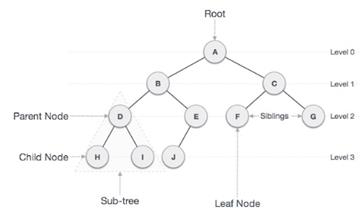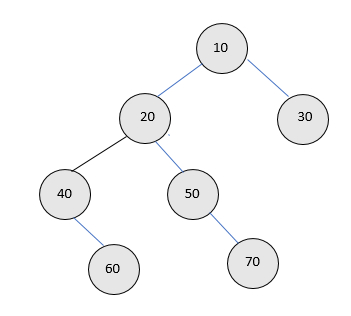# Count half nodes in a Binary tree (Iterative and Recursive) in C++

We are given a binary tree and the task is to calculate the count of half nodes available in a binary tree using iterative and recursive approach. Half nodes are those nodes who have only one child and another child is null. Note that in half nodes we don't consider leaf nodes.

Binary Tree is a special data structure used for data storage purposes. A binary tree has a special condition that each node can have a maximum of two children. A binary tree has the benefits of both an ordered array and a linked list as search is as quick as in a sorted array and insertion or deletion operation are as fast as in linked list. Non-leaf nodes are also known as parent nodes as they have more than 0 child and less than two children.

Structure of a binary tree is given below −## For Example

InputOutput − count is 2

Explanation − in the given tree there are 2 nodes i.e. 40 and 50 with exactly one child or half nodes other nodes may have two children or no child.

## Iterative

### Approach used in the below program is as follows

• Create a structure of a node containing a data part, left pointer and right pointer.

• Create a function to insert the nodes in a binary tree.

• Create a function to count the half nodes.

• Inside a function, check IF !node then return as there is no node in a tree.

• Declare a temporary variable count to store the count of half nodes

• Create a queue type variable let’s say qu

• Push the nodes in a queue as qu.push(node)

• Loop while !qu.empty()

• Create a temporary variable let’s say temp of Node type and initialize it with queue.front()

• Pop the elements using qu.pop()

• Check IF (!temp-> leftAND temp-> right) OR (temp->left AND !temp->right) then increment the count by 1

• Check IF (temp->left != NULL) then perform qu.push(temp->left)

• Return the count

• Print the result.

## Example

Live Demo

// Program to count half nodes in a Binary Tree
#include <iostream>
#include <queue>
using namespace std;
struct Node{
int data;
struct Node* left, *right;
};
// Function to get the count of half Nodes
int halfcount(struct Node* node){
// If tree is empty
if (!node)
return 0;
int result = 0; // Initialize count of half nodes
// Do level order traversal starting from root
queue<Node *> myqueue;
myqueue.push(node);
while (!myqueue.empty()){
struct Node *temp = myqueue.front();
myqueue.pop();
if ((!temp->left && temp->right) || (temp->left && !temp->right)){
result++;
}
if (temp->left != NULL){
myqueue.push(temp->left);
}
if (temp->right != NULL){
myqueue.push(temp->right);
}
}
return result;
}
/* To allocate new Node with the given data and NULL left
and right pointers. */
struct Node* newNode(int data){
struct Node* node = new Node;
node->data = data;
node->left = node->right = NULL;
return (node);
}
int main(void){
struct Node *root = newNode(10);
root->left = newNode(20);
root->right = newNode(30);
root->left->left = newNode(40);
root->left->right = newNode(50);
root->left->left->right = newNode(60);
root->left->right->right = newNode(70);
cout <<"count is: "<<halfcount(root);
return 0;
}

## Output

If we run the above code we will get the following output −

count is: 2

## Recursive

### Approach used in the below program is as follows

• Create a structure of a node containing a data part, left pointer and right pointer.

• Create a function to insert the nodes in a binary tree.

• Create a function to count the half nodes.

• Inside a function, check IF !node then return as there is no node in a tree.

• Declare a temporary variable count to store the count of half nodes

• Check IF (root -> left = NULL AND root->right != NULL) OR (root -> left != NULL AND root->right == NULL) then increment the count by 1

• Set count = count + recursive_call_to_this_function(root->left) + recursive_call_to_this_function(root->right)

• Return the count

• Print the result.

## Example

Live Demo

// Recursive program to count half nodes
#include <bits/stdc++.h>
using namespace std;
// A binary tree Node has data, pointer to left
// child and a pointer to right child
struct Node{
int data;
struct Node* left, *right;
};
int halfcount(struct Node* root){
if (root == NULL)
return 0;
int result = 0;
if ((root->left == NULL && root->right != NULL) || (root->left != NULL && root->right ==
NULL)){
result++;
}
result += (halfcount(root->left) + halfcount(root->right));
return result;
}
/* to allocate a new Node with the given data and NULL left
and right pointers. */
struct Node* newNode(int data){
struct Node* node = new Node;
node->data = data;
node->left = node->right = NULL;
return (node);
}
int main(){
struct Node *root = newNode(10);
root->left = newNode(20);
root->right = newNode(30);
root->left->left = newNode(40);
root->left->right = newNode(50);
root->left->left->right = newNode(60);
root->left->right->right = newNode(70);
cout <<"count is: "<<halfcount(root);
return 0;
}

## Output

If we run the above code we will get the following output −

count is: 2